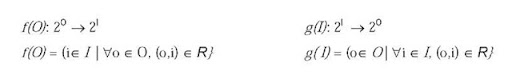# Algorithms for Association Rule Mining (Artificial Intelligence)

## INTRODUCTION

Association Rule Mining (ARM) is one of the important data mining tasks that has been extensively researched by data-mining community and has found wide applications in industry. An Association Rule is a pattern that implies co-occurrence of events or items in a database. Knowledge of such relationships in a database can be employed in strategic decision making in both commercial and scientific domains.

A typical application of ARM is market basket analysis where associations between the different items are discovered to analyze the customer’s buying habits. The discovery of such associations can help to develop better marketing strategies. ARM has been extensively used in other applications like spatial-temporal, health care, bioinformatics, web data etc (Hipp J., Guntzer U., Nakhaeizadeh G. 2000).

An association rule is an implication of the form X ^ Y where A” and Y are independent sets of attributes/items. An association rule indicates that if a set of items Xoccurs in a transaction record then the set of items Yalso occurs in the same record. X is called the antecedent of the rule and Yis called the consequent of the rule. Processing massive datasets for discovering co-occurring items and generating interesting rules in reasonable time is the objective of allARM algorithms. The task of discovering co-occurring sets of items cannot be easily accomplished using SQL, as a little reflection will reveal. Use of ‘Count’ aggregate query requires the condition to be specified in the where clause, which finds the frequency of only one set of items at a time. In order to find out all sets of co-occurring items in a database with n items, the number of queries that need to be written is exponential in n. This is the prime motivation for designing algorithms for efficient discovery of co-occurring sets of items, which are required to find the association rules.

In this article we focus on the algorithms for association rule mining (ARM) and the scalability issues in ARM. We assume familiarity of the reader with the motivation and applications of association rule mining

## BACKGROUND

Let I = {i, i,.., i} denote a set of items and Ddenote a database of ^transactions. A typical transaction Te D may contain a subset X of the entire set of items I and is associated with a unique identifier TID. An item-set is a set of one or more items i.e. Xis an item-set if Xc I. A k-item-set is an item-set of cardinality k. A transaction is said to contain an item-set Xif Xc T. Support of an item set X, also called Coverage is the fraction of transactions that contain X. It denotes the probability that a transaction contains X.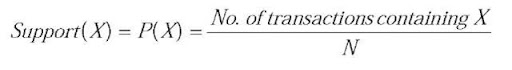An item-set having support greater than the user specified support threshold (ms) is known as frequent item-set.

An association rule is an implication of the form X ^Y [Support, Confidence] where Xc I, Yc /and Xn Y =0, where Support and Confidence are rule evaluation metrics. Support of a rule X ^ Y in D is ‘S” if S% of transactions in D contain Xu Y. It is computed as: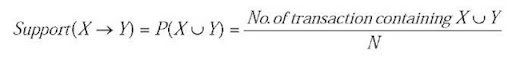Support indicates the prevalence of a rule. In a typical market basket analysis application, rules with very low support values represent rare events and are likely to be uninteresting or unprofitable. Confidence of a rule measures its strength and provides an indication of the reliability of prediction made by the rule. A rule X ^ Y has a confidence ‘C” in D if C % of transactions in D that contain X, also contain Y. Confidence is computed, as the conditional probability of Yoccuring in a transaction, given Xis present in the same transaction, i.e.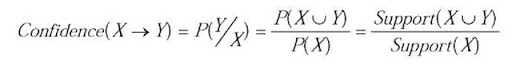A rule generated from frequent item-sets is strong if its confidence is greater than the user specified confidence threshold (mc). Fig. 1 shows an example database of five transactions and shows the computation of support and confidence of a rule.

The objective of Association Rule Mining algorithms is to discover the set of strong rules from a given database as per the user specified ms and mc thresholds. Algorithms for ARM essentially perform two distinct tasks: (1) Discover frequent item-sets. (2) Generate strong rules from frequent item-sets.

The first task requires counting of item-sets in the database and filtering against the user specified threshold (ms). The second task of generating rules from frequent item-sets is a straightforward process of generating subsets and checking for the strength. We describe below the general approaches for finding frequent item-sets in association rule mining algorithms. The second task is trivial as explained in the last section of the article.

## APPROACHES FOR GENERATING FREQUENT ITEM-SETS

If we apply a brute force approach to discover frequent item-sets, the algorithm needs to maintain counters for all 2″ -1 item-sets. For large values of n that are common in the datasets being targeted for mining, maintaining such large number of counters is a daunting task. Even if we assume availability of such large memory, indexing of these counters also presents a challenge. Data mining researchers have developed numerous algorithms for efficient discovery of frequent item-sets.

The earlier algorithms for ARM discovered all frequent item-sets. Later it was shown by three independent groups of researchers (Pasquier N., Bastide Y., Taouil R. & Lakhal L. 1999), (Zaki M.J. 2000), (Stumme G., 1999), that it is sufficient to discover frequent closed item-sets (FCI) instead of all frequent item-sets (FI). FCI are the item-sets whose support is not equal to the support of any of its proper superset. FCI is a reduced, complete and loss less representation of frequent item-sets. Since FCI are much less in number than FI, computational expense for ARM is drastically reduced.

Figure 2 summarizes different approaches used for ARM. We briefly describe these approaches.

Discovery of Frequent Item-Sets

Level-Wise Approach

Level wise algorithms start with finding the item-sets of cardinality one and gradually work up to the frequent item-sets of higher cardinality. These algorithms use anti-monotonic property of frequent item-sets according to which, no superset of an infrequent item-set can be frequent.

Figure 1. Computation of support and confidence of a rule in an example database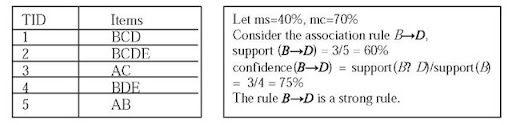Figure 2. Approaches for ARM algorithms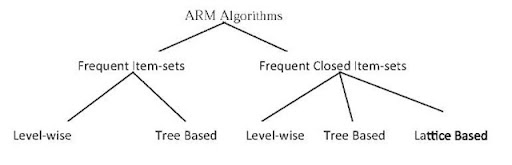Agarwal et al. (Agarwal, R., Imielinski T., & Swami A. 1993), (Agarwal, R., & Swami A., 1994) proposed Apriori algorithm, which is the most popular iterative algorithm in this category. It starts, with finding the frequent item-sets of size one and goes up level by level, finding candidate item-sets of size k by joining item-sets of size k-1. Two item-sets, each of size k-1 join to form an item-set of size k if and only if they have first k-2 items common. At each level the algorithm prunes the candidate item-sets using anti-monotonic property and subsequently scans the database to find the support of pruned candidate item-sets. The process continues till the set of frequent item-sets is nonempty. Since each iteration requires a database scan, maximum number of database scans required is same as the size of maximal item-set. Fig. 3 and Fig 4 gives the pseudo code of Apriori algorithm and a running example respectively.

Two of the major bottlenecks in Apriori algorithm are i) number of passes and ii) number of candidates generated. The first is likely to cause I/O bottleneck and the second causes heavy load on memory and CPU usage. Researchers have proposed solutions to these problems with considerable success. Although detailed discussion of these solutions is beyond the scope of this article, a brief mention is necessary.

Hash techniques reduce the number of candidates by making a hash table and discarding a bucket if it has support less than the ms. Thus at each level memory requirement is reduced because of smaller candidate set. The reduction is most significant at lower levels. Maintaining a list of transaction ids for each candidate set reduces the database access. Dynamic Item-set Counting algorithm reduces the number of scans by counting candidate sets of different cardinality in a single scan (Brin S., Motwani R., Ullman J.D., & Tsur S. 1997). Pincer Search algorithm uses a bi-directional strategy to prune the candidate set from top (maximal) and bottom (1-itemset) (Lin D. & Kedem Z.M. 1998). Partitioning and Sampling strategies have also been proposed to speed up the counting task. An excellent comparison of Apriori algorithm and its variants has been given in (Hipp J., Guntzer U., Nakhaeizadeh G. 2000).

### Tree Based Algorithms

Tree based algorithms have been proposed to overcome the problem of multiple database scans. These algorithms compress (sometimes lossy) the database into a tree data structure and reduce the number of database scans appreciably. Subsequently the tree is used to mine for support of all frequent item-sets.

Set-Enumeration tree used in Max Miner algorithm (Bayardo R.J. 1998) orders the candidate sets while searching for maximal frequent item-sets. The data structure facilitates quick identification of long frequent item-sets based on the information gathered during each pass. The algorithm is particularly suitable for dense databases with maximal item-sets of high cardinality.

Han et. al. (Han, J., Pei, J., & Yin, Y. 2000) proposed Frequent Pattern (FP)-growth algorithm which performs a database scan and finds frequent item-sets of cardinality one. It arranges all frequent item-sets in a table (header) in the descending order of their supports. During the second database scan, the algorithm constructs in-memory data structure called FP-Tree by inserting each transaction after rearranging it in descending order of the support. A node in FP-Tree stores a single attribute so that each path in the tree represents and counts the corresponding record in the database. A link from the header connects all the nodes of an item. This structural information is used while mining the FP-Tree. FP-Growth algorithm recursively generates sub-trees from FP-Trees corresponding to each frequent item-set.

Figure 3. Apriori algorithm

Input: Database D of N transactions

ms, mc

Output: Set L of frequent item-sets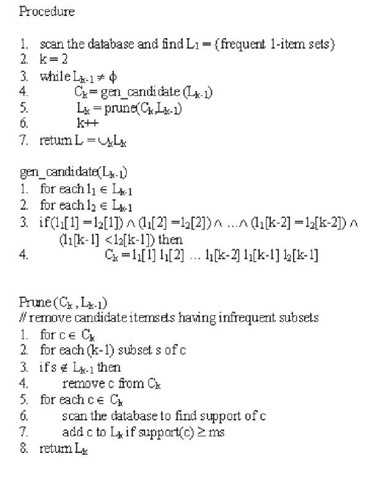Coenen et. al. (Coenen F., Leng P., & Ahmed S. 2004) proposed Total Support Tree (T-Tree) and Partial Support Tree (P-Tree) data structures which offer significant advantage in terms of storage and execution. These data structures are compressed set enumeration trees and are constructed after one scan of the database and stores all the item-sets as distinct records in database.

## Discovery of Frequent Closed Item-Sets

### Level Wise Approach

Pasquier et. al. (Pasquier N., Bastide Y., Taouil R. & Lakhal L. 1999) proposed Close method to find

Frequent Closed Item-sets (FCI). This method finds closures based on Galois closure operators and computes the generators. Galois closure operator h(X) for some Xc lis defined as the intersection of transactions in D containing item-set X. An item-set X is a closed item-set if and only if h(X) = X. One of the smallest arbitrarily chosen item-set p, such that h(p) = X is known as generator of X.

Close method is based on Apriori algorithm. It starts from 1- item-sets, finds the closure based on Galois closure operator, goes up level by level computing generators and their closures (i.e. FCI) at each level. At each level, candidate generator item-sets of size k are found by joining generator item-sets of size k-1 using the combinatorial procedure used in Apriori algorithm. The candidate generators are pruned using two strategies i) remove candidate generators whose all subsets are not frequent ii) remove the candidate generators if closure of one of its subsets is superset of the generator. Subsequently algorithm finds the support of pruned candidate generator. Each iteration requires one pass over the database to construct the set of FCI and count their support.

Figure 4. Running example of apriori algorithm for finding frequent itemsets (ms = 40%)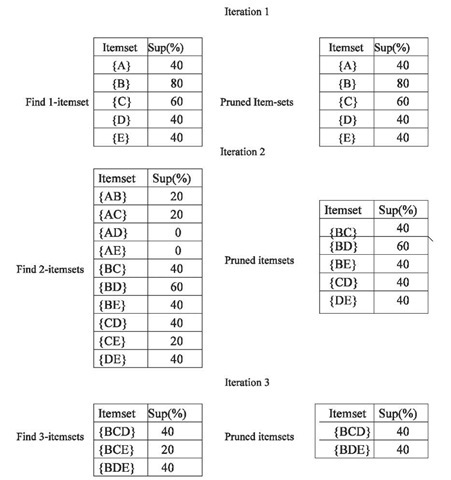Iteration 4: No candidate item-sets

### Tree Based Approach

Wang et. al. (Wang J., Han J. & Pei J. 2003) proposed Closet+ algorithm to compute FCI and their supports using FP-tree structure. The algorithm is based on divide and conquers strategy and computes the local frequent items of a certain prefix by building and scanning its projected database.

### Concept Lattice Based Approach

Concept lattice is a core structure of Formal Concept Analysis (FCA). FCA is a branch of mathematics based on Concept and Concept hierarchies. Concept (A,B) is defined as a pair of set of objects A (known as extent) and set of attributes B (known as intent) such that set of all attributes belonging to extent A is same as B and set of all objects containing attributes of intent B is same as A. In other words, no object other than objects of set A contains all attributes of B and no attribute other than attributes in set B is contained in all objects of set A. Concept lattice is a complete lattice of all Concepts. Stumme G., (1999) discovered that intent

Exhibit A. add extent {all transactions} in the list of extents For each item i e I for each set X in the list of extents find Xn {set of transactions containing i} include in the list of extents if not included earlier

EndFor

B of the Concept (A,B) represents the closed item-set, which implies that all algorithms for finding Concepts can be used to find closed item-sets. Kuznetsov S.O., & Obiedkov S.A. (2002) provides a comparison of performance of various algorithms for concepts. The naive method to compute Concepts, proposed by Ganter is given in Exhibit A.

This method generates all the Concepts i.e. all closed item-sets. Closed item-sets generated using this method in example 1 are {A},{B} ,{C},{A,B},{A,C},{B,D},{B, C,D}, {B,D,E}, {B,C,D,E}. Frequent Closed item-sets are {A} ,{B},{C},{B,D},{B,C,D},{B,D,E}.

Concept lattice for frequent closed item-sets is given in Figure 5.

### Generating Association Rules

Once all frequent item-sets are known, association rules can be generated in a straightforward manner by finding all subsets of an item-sets and testing the strength (Han J., & Kamber M., 2006). The pseudo code for this algorithm is given in Exhibit B.

Based on the above algorithm, strongrules generated from frequent item-set BCD in Example 1 are: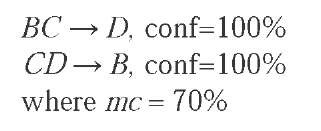There are two ways to find association rules from frequent closed item-sets:

i) compute frequent item-sets from FCI and then find the association rules

ii) generate rules directly using FCI.

Close method uses the first approach, which generates lot of redundant rules while method proposed by Zaki (Zaki M.J., 2000), (Zaki, M.J., & Hsiao C., J., 2005) uses the second approach and derives rules directly from the Concept lattice. The association rules thus derived are non-redundant rules. For example, set of strong rules generated using Close method in Example 1 is {BC ^ D,CD ^B,D ^B,E ^ B,E ^D,E ^ BD, BE ^D,DE ^B}. For the same example, set of non-redundant strong rules generated using Concept Lattice approach is {D ^B, E ^ BD, BC ^ D, CD ^ B}. We can observe here that all rules can be derived from the reduced non-redundant set of rules.

Figure 5. Concept lattice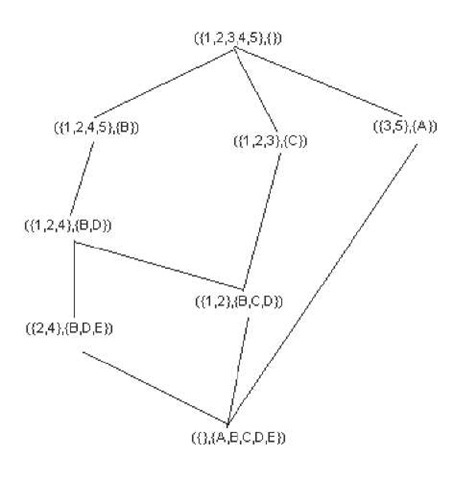### Scalability issues in Association Rule Mining

Scalability issues in ARM have motivated development of incremental and parallel algorithms. Incremental algorithms for ARM preserve the counts of selective item-sets and reuse this knowledge later to discover frequent item-sets from augmented database. Fast update algorithm (FUP) is the earliest algorithm based on this idea. Later different algorithms are presented based on sampling (Hipp J., Guntzer U., & Nakhaeizadeh G., 2000).

Parallel algorithms partition either the dataset for counting or the set of counters, across different ma-

### Exhibit B.

For each frequent item-set I, generate all non-empty subsets of I For every non-empty subset s of I,

Output the rule s ^ (I-s) if support(I) / support (s) >= mc EndFor chines to achieve scalability (Hipp J., Guntzer U., & Nakhaeizadeh G., 2000). Algorithms, which partition the dataset exchange counters while the algorithms, which partition the counters, exchange datasets incurring high communication cost.

## FUTURE TRENDS

Discovery of Frequent Closed Item-sets (FCI) is a big lead in ARM algorithms. With the current growth rate of databases and increasing applications of ARM in various scientific and commercial applications we envisage tremendous scope for research in parallel, incremental and distributed algorithms for FCI. Use of lattice structure for FCI offers promise of scalability. On line mining on streaming datasets using FCI approach is an interesting direction to work on.

## CONCLUSION

The article presents the basic approach for Association Rule Mining, focusing on some common algorithms for finding frequent item-sets and frequent closed item-sets. Various approaches have been discussed to find such item-sets. Formal ConceptAnalysis approach for finding frequent closed item-sets is also discussed. Generation of rules from frequent items-sets and frequent closed item-sets is briefly discussed. The article addresses the scalability issues involved in various algorithms.

## KEY TERMS

Association Rule: An Association rule is an implication of the form X^Y where X c I, Yc I and X^Y =0, I denotes the set of items.

Data Mining: Extraction of interesting, non-trivial, implicit, previously unknown and potentially useful information or patterns from data in large databases.

Formal Concept: A formal context K = (G,M,I) consists of two sets G (objects) and M (attributes) and a relation I between G and M. For a set AcG of objects A ‘={meM / gIm for all geA} (the set of all attributes common to the objects in A). Correspondingly, for a set B of attributes we define B’ = {geG / gIm for all meB} (the set of objects common to the attributes in B).

A formal concept of the context (G,M,I) is a pair (A,B) with AcG,BcM, A’=B and B’=A

A is called the extent and B is the intent of the concept (A,B).

Frequent Closed Item-Set: An item-set Xis a closed item-set if there exists no item-set X’ such that:

i. X’ is a proper superset of X,

ii. Every transaction containing X also contains X’.

A closed item-set Xis frequent if its support exceeds the given support threshold.

Galois Connection: Let D = (O,I,R) be a data mining context where O and I are finite sets of objects (transactions) and items respectively. R c O x I is a binary relation between objects and items. For O c O, and I c I, we define as shown in Exhibit C.

f(O) associates with O the items common to all objects o e O and g(I) associates with I the objects related to all items i e I. The couple of applications (f,g) is a Galois connection between the power set of O (i.e. 2O) and the power set of I (i.e. 2′).

The operators h = f o g in 2′ and h’=g o fin 2o are Galois closure operators. An item-set C c I from D is a closed item-set iff h(C) = C.

Generator Item-Set: A generator p of a closed item-set c is one of the smallest item-sets such that h(p) = c.

Non-Redundant Association Rules: Let R denote i the rule X^XJ, where Xj X2c I. Rule R1 is more general than rule R2 provided R2 can be generated by adding additional items to either the antecedent or consequent of R1. Rules having the same support and confidence as more general rules are the redundant association rules. Remaining rules are non-redundant rules.

### Exhibit C.